The SSM Procedure

TREND Statement

• TREND name ( type ) <options>;

The TREND statement defines a term in the model that follows a stochastic pattern of a certain predefined type. The options in the TREND statement enable you to specify a wide variety of commonly used stochastic patterns. Each TREND statement in effect stands for a special pair of STATE and COMPONENT statements. You can specify more than one TREND statement. Each separate TREND statement defines a component that is assumed to be independent of all other component specifications in the model. Very often the TREND statement is used to specify a component that captures the time-varying level of the data. However, in many cases it is also used to define components of a more general nature; for example, it can be used to define a noise component that follows a stationary ARMA model.

You can refer to the state that is associated with a TREND statement by appending the string "_state_" to the end of its name. For example, name_state_ is the state that is associated with a trend named name. You can use name_state_ in a COMPONENT statement to define a linear combination of its elements. The estimate of this linear combination can then be printed or output to a data set. The nominal dimension of name_state_ is taken to be 1, or the number of variables in the list that is specified in the CROSS= option in the TREND statement that is used to define name (see Example 27.4 for an example of such use of the COMPONENT statement).

Some of these trend specifications are applicable to all the data types—that is, they can be used for both regular data types and irregular data types, whereas the others require that the data be regular or regular with replication. Of course, the trend specification is only part of the overall model specification. Therefore, the other parts of the model can imply additional constraints on the data type.

Table 27.3 lists the available trend models and their data requirements. The type column shows the admissible keywords that signify the particular trend type. For brevity, the Data Type column groups the data types regular and regular with replication into one category: regular. For more information about these trend models, see the section Predefined Trend Models.

Table 27.3: Summary of Trend Types

type

Data Type

Description

Parameters

ARIMA(P=integer D=integer …)

Regular

ARIMA model specification

AR and MA coefficients,

and the error variance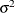DLL

Regular

Damped local linear

Level and slope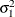,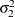,

damping factorLL

Regular

Local linear

Level and slope,RW

Regular

Random walk

LevelDECAY

Irregular

A type of decay pattern

Level, decay rateDECAY(OU)

Irregular

Ornstein-Uhlenbeck decay pattern

Level, decay rateGROWTH

Irregular

A type of growth pattern

Level, growth rateGROWTH(OU)

Irregular

Ornstein-Uhlenbeck growth pattern

Level, growth ratePS(order)

Irregular

Polynomial spline of a given order

LevelThe keyword specification of different trend types, except possibly the ARIMA trend, is quite simple. For example, the following statement specifies polySpline as a trend of the type second-order polynomial spline:

      trend polySpline(ps(2));


Similarly, the following statement defines dampedTrend as a damped local linear trend:

      trend dampedTrend(dll) slopevar=x;


The variance parameter that governs the slope equation of this trend type is given by a variable x, which must be defined elsewhere in the program. The other parameters that define dampedTrend are left unspecified (and are estimated by using the data).

The ARIMA trend specification permits specification of trends that follow an ARIMA(p,d,q)(P,D,Q)model. The specification of ARIMA models requires some notation, which is explained first.

Let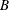denote the backshift operator—that is, for any sequence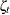,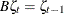. The higher powers ofrepresent larger shifts (for example,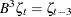). A random sequencefollows an ARIMA(p,d,q)(P,D,Q)model with nonseasonal autoregressive order p, seasonal autoregressive order P, nonseasonal differencing order d, seasonal differencing order D, nonseasonal moving average order q, and seasonal moving average order Q if it satisfies the following difference equation, which is specified in terms of the polynomials in the backshift operator, where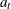is a white noise sequence and s is the season length: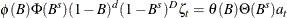The polynomials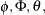and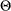are of orders p, P, q, and Q, respectively, which can be any nonnegative integers. The season length s must be a positive integer. For example,satisfies an ARIMA(1,0,1) model (that is,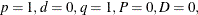and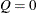) if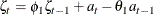for some coefficients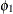and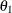and a white noise sequence. Similarly,satisfies an ARIMA(0,1,1)(0,1,1)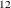model if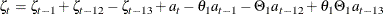for some coefficientsand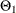and a white noise sequence. An ARIMA process is zero-mean, stationary, and invertible if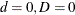, and the defining polynomialsandhave all their roots outside the unit circle—that is, their absolute values are strictly larger than 1.0. It is assumed that the coefficients of the polynomialsandare constrained so that the stationarity and invertibility conditions are satisfied. The unknown coefficients of these polynomials become part of the model parameter vector that is estimated by using the data. The general form of the ARIMA trend specification is as follows:

• ARIMA(<P=integer> <D=integer> <Q=integer> <SP=integer> <SD=integer> <SQ=integer> <S=integer> )

By default, the different orders are equal to 0 and the season length is equal to 1. The following examples illustrate a few different ARIMA trend specifications. The following statement defines ima as an integrated moving average trend:

      trend ima(arima(d=1 q=1));


The following statement defines airTrend as a trend that satisfies the well-known airline model (ARIMA(0,1,1)(0,1,1)12 model) for monthly seasonal data:

      trend airTrend(arima(d=1 q=1 sd=1 sq=1 s=12));


The following statement defines arma11 as a zero-mean ARMA(1,1) trend with autoregressive parameter fixed to 0.1:

      trend arma11(arima(p=1 q=1)) ar=0.1;


For an example of the use of the ARIMA trend specification, see Example 27.6.

You can use the following options in the TREND statement to specify the trend parameters and to request printing of the trend estimates. In addition, you can create a custom combination of a given trend type by specifying the CROSS= option to create a more general trend. For an example of using the CROSS= option, see the section Getting Started: SSM Procedure and the discussion of the second model in Example 27.4. You can also check for the unexpected changes in the trend component by using the CHECKBREAK option.

AR=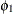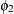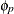lists the values of the coefficients of the nonseasonal autoregressive polynomial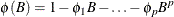where the order p is specified in the ARIMA trend specification. The coefficients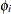must define a stationary autoregressive polynomial.

CHECKBREAK<( ELEMENTWISE | OVERALL)>

turns on the checking of breaks for this trend component. The ELEMENTWISE suboption requests the elementwise checking of any unexpected change in the state subsection that is associated with the trend component. The OVERALL suboption requests a similar check for the entire state subsection—that is, in this case the change is measured as a multidimensional change. The ELEMENTWISE suboption is the default. Unless the PRINT=BREAKDETAIL option is specified, only a summary of the most significant breaks is produced. If the PRINT=BREAKDETAIL is specified, tables that contain the break significance statistics at every distinct time point are produced—one for the ELEMENTWISE suboption and one for the OVERALL suboption. If the CROSS= option is specified and the CROSS= list contains more than one variable, the OVERALL suboption considers subsections that are associated with each CROSS= variable separately. For more information about the structural break detection process, see the section Structural Breaks in the State Evolution.

CROSS=(var1, var2, ...)
CROSS(MATCHPARM)=(var1, var2, ...)

creates a linear combination of one or more independent trend components that is based on the variables in the list. If the parameters of the trend are specified by options such as the LEVELVAR= option or the PHI= option, these parameters are shared by these constituent trends. For example, suppose that the CROSS= list contains two variables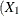and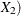and the trend specification is of the type RW. The effect of CROSS=(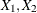) is to create a component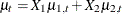, where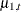and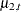are two independent random walk trends. Moreover, if the random walk trend specification uses the LEVELVAR= option to specify the variance parameter,andshare the same variance parameter; otherwise, two separate variance parameters are assigned to these random walks. If the second form of the CROSS option, CROSS(MATCHPARM)=, is used, then the constituent trends share all the relevant parameters no matter how they are specified. The CROSS= option is useful for a variety of situations. For example, suppose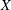is an indicator variable that is 1 before a certain time point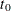and 0 thereafter. Then CROSS=(X) has the effect of turning off the trend component after time. Similarly, suppose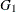and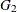are indicators for gender—for example,= (GENDER=1) and= (GENDER=0) for male and female cases, respectively. Then CROSS=(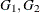) results in separate trends according to the gender. The variables in the CROSS= list must be free of unknown parameters.

The CROSS= option can be computationally expensive; computationally it is equivalent to specifying as many separate trends as the number of variables in the specified list.

LEVELVAR=variable | number

specifies the disturbance variance parameter for all the trend types. For trend types LL and DLL, this option specifies. Any nonnegative value, including 0, is permissible. If variable contains unknown parameters, they are estimated from the data. Similarly, if the LEVELVAR= option is not specified,is estimated from the data.

MA=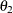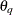lists the values of the coefficients of the nonseasonal moving average polynomial,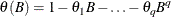where the order q is specified in the ARIMA trend specification. The coefficients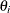must define an invertible moving average polynomial.

NODIFFUSE

causes the diffuse elements in the initial state of the state subsection underlying the trend component to be treated as nondiffuse. This option is applicable to all trend types except ARIMA. For the ARIMA trend type, this option is ignored even if the nonseasonal or seasonal differencing orders are nonzero. The diffuse elements are assumed to be independent, zero-mean, Gaussian variables. Their variances become part of the parameter vector and are estimated by using the data. This option is useful for creating a trend component that can be interpreted as a deviation from an overall trend component (with diffuse initialization), which is defined separately.

PHI=variable | number

specifies the value offor trend types DLL, DECAY, DECAY(OU), GROWTH, and GROWTH(OU). For the type DLL, the specified value must be between 0.0 and 1.0. For types DECAY and DECAY(OU),must be strictly negative. For types GROWTH and GROWTH(OU),must be strictly positive. If variable contains unknown parameters, they are estimated from the data. Similarly, if the PHI= option is not specified,is estimated from the data.

PRINT=BREAKDETAIL | COV | COV1 | FILTER | SMOOTH | T
PRINT= (<BREAKDETAIL> <COV> <COV1> <FILTER> <SMOOTH> <T> )

requests printing of the respective system matrices of the state equation that underlies the specified trend, the printing of its filtered and smoothed estimates, and the printing of the break statistics at each distinct time point. For the BREAKDETAIL suboption to have any effect, the CHECKBREAK option must be turned on. If any of these matrices are time-varying, the matrix that corresponds to the first time instance is printed.

SAR=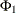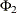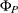lists the values of the coefficients of the seasonal autoregressive polynomial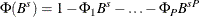where the order P is specified by using the SP= option in the ARIMA trend specification and the season length s is specified in the S= option. The coefficients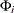must define a stationary autoregressive polynomial.

SMA=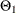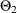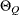lists the values of the coefficients of the seasonal moving average polynomial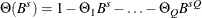where the order Q is specified by using the SQ= option in the ARIMA trend specification and the season length s is specified in the S= option. The coefficients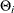must define an invertible moving average polynomial.

SLOPEVAR=variable | number

specifies the second disturbance variance parameter,, for trend types LL and DLL. Any nonnegative value, including 0, is permissible. If variable contains unknown parameters, they are estimated from the data. Similarly, if the SLOPEVAR= option is not specified,is estimated from the data.